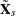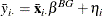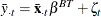# The PANEL Procedure

### Between Estimators

The between-groups estimator is the regression of the cross section means ofon the cross section means of. In other words, you fit the following regression:The between-time-periods estimator is the regression of the time means ofon the time means of. In other words, you fit the following regression:In either case, the error is assumed to be normally distributed with mean zero and a constant variance.